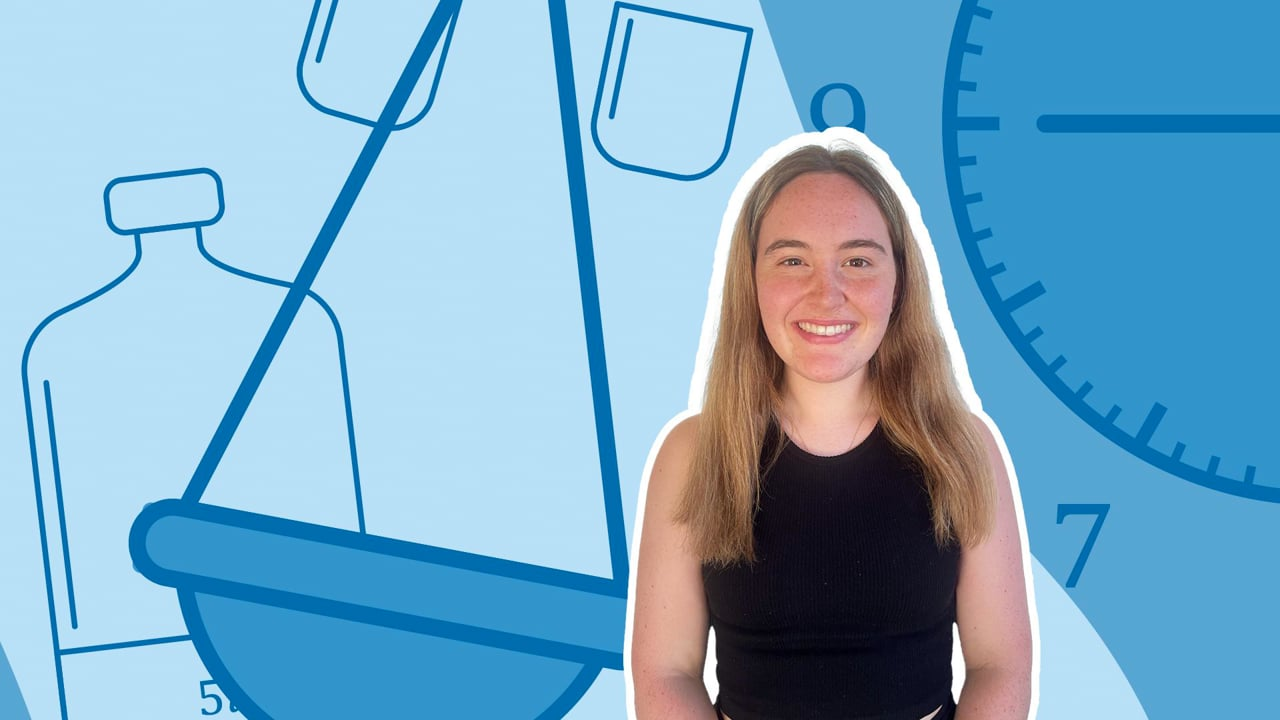Chapter OverviewMaths

Number and place value

Multiplication and division

Fractions

Measurement

Geometry - properties of shapes

Statistics

Maths

# Length: Comparing, adding and subtracting0%

Summary

# Length: Comparing, adding and subtracting

## ​​In a nutshell

Length is how you describe how long an object is compared to another. You can compare the sizes of objects using length and for small enough objects you can use a ruler to measure this.

## Comparing length

Bigger objects have bigger lengths, so a $20cm$​ fork is bigger than a $5cm$​ eraser. Remember that you can only compare lengths if they have the same unit; an $11cm$ pencil is not longer than a $2m$​ table! Use this equation to convert between units:

$100cm = 1m = 0.001km$

#### procedure

 1 Make sure the units are the same. 2 Identify what the question is asking you:"Find the total length of ..." means summing the lengths."How much longer is ..." means subtracting one length from the other. 3 Convert the answer back into appropriate units (if required).

##### Example

How much longer is a $1.2m$​ table compared to a $65cm$​ chair?

$1.2m = 120cm$

$120 - 65 = \underline{55cm}$

FAQs

• Question: What happens when you use units of different length to measure with?

Answer: The size of the unit used to measure something affects the measurement. If you measure the same quantity with different units, it will take more of the smaller unit and fewer of the larger unit to express the measurement.

• Question: Which units of length are appropriate when measuring longer objects?

Answer: Objects larger than 10 mm but smaller than a metre should be measured using centimetres, and objects larger than a metre should be measured using metres. Very large distances should be measured in units of kilometres.

• Question: What units are used to measure length?

Answer: A kilometre (km), metre (m) and centimetre (cm) are the commonly used units of length.

Theory

Exercises